## A 460 W heating unit is designed to operate with an applied potential difference of 120 V (a) By what percentage will its heat output drop i

Question

A 460 W heating unit is designed to operate with an applied potential difference of 120 V (a) By what percentage will its heat output drop if the applied potential difference drops to 110 V? Assume no change in resistance. %(b) If you took the variation of resistance with temperature into account, would the actual drop in heat output be larger or smaller than that calculated in (a)?

in progress 0
2 months 2021-07-29T16:02:39+00:00 1 Answers 3 views 0

(a) = -0.16%

(b) = smaller

Explanation:

given

power = 460 W

potential difference = 120 V

(a) what percentage will   its heat output drop if the applied potential difference drops to 110 V ?

we know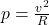…………………(i)

we need to find change in power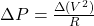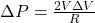…………..(ii)

from equations we get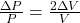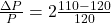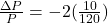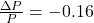(b)

if we increase temperature resistance will increase and decrease with decrease in temperature and we know power is inversely proportional to resistance so if potential decrease and it would cause drop in power

and due to this increment of heating power resistance will decrease so actual drop in the power would  be smaller